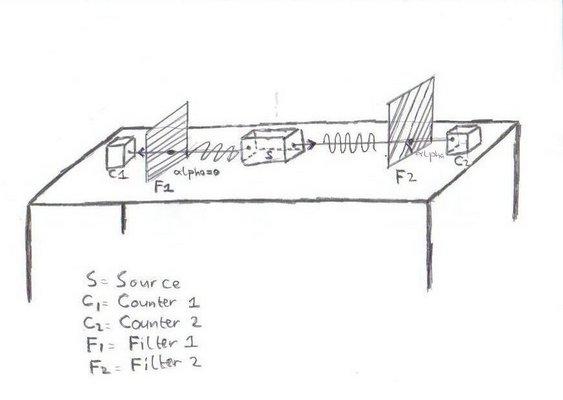## Entangled Photon PairThe assumption is:
• That the left photon moves towards the left and is polarised in the horizontal plane .
• That the right photon moves towards the right and is polarised in a vertical plane .
• That the angle of the left filter is zero.
What the sketch shows is, that when the angle "alpha" of the right filter
• is 90 degrees that an entangled photon pair goes to both filters and will be counted twice and simultaneous
• is 0 degrees that from an entangled photon pair only the left photon goes through a filter and will be counted once
• is 45 degrees that from an entangled photon pair at least the left photon will be counted and that there is a 50% chance that also the right photon (will go through a filter) and will be counted